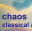# Turbulence and what to do about it

## FANTOM Study Week, May 2005 - Lecture notes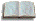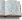## Chapter 1 - Overture

An overview of the course. Skim quickly through - parts will make sense only in the rear view mirror, do not worry that you do not understand them yet.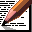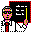appendix A - You might also want to read about the history of the subject.## Chapter 2 - Flows

A recapitulation of basic notions of dynamics. The reader familiar with the dynamics on the level of an introductory graduate nonlinear dynamics course can safely skip this material, hop to chapter 9: Transporting densities. Just in case - make sure you understand

section 2.4: "Infinite-dimensional flows".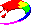## Chapter 3 - Maps

Discrete time dynamics arises by considering sections of a continuous flow. There are also many settings in which dynamics is discrete, and naturally described by repeated applications of a map.## Chapter 4 - Local stability

Review of basic concepts of local dynamics: local linear stability for flows and maps.

Skip section 4.4.1.## Chapter 8 - Cycle stability

Cycle eigenvalues are flow invariants.

Skip sections 8.3, 8.4 and 8.5.## Chapter 9 - Transporting densities

A first attempt to move the whole phase space around - natural measure and fancy operators.

Skip section 9.5.## Chapter 10 - Averaging

On the necessity of studying the averages of observables in chaotic dynamics. Formulas for averages are cast in a multiplicative form that motivates the introduction of evolution operators.

Skip sections 10.3 and 10.4.## Chapter 11 - Qualitative dynamics, for pedestrians

Qualitative dynamics of simple stretching and mixing flows; Smale horseshoes and symbolic dynamics. The topological dynamics is incoded by means of transition matrices/Markov graphs.

Skip sections 11.1.1 and 11.5.## Chapter 13 - Counting, for pedestrians

You learn here how to count and describe itineraries. While computing the topological entropy from transition matrices/Markov graphs, we encounter our first zeta function.

Skip sections 13.4.1 and 13.5.## Chapter 14 - Trace formulas

If there is one idea that one should learn about chaotic dynamics, it happens in this chapter: the (global) spectrum of the evolution is dual to the (local) spectrum of periodic orbits. The duality is made precise by means of trace formulas.

Skip sections 14.2.1, 14.3 and 14.4.## Chapter 15 - Spectral determinants

We derive the spectral determinants, dynamical zeta functions.

Skip sections 15.2, 15.3.1, 15.3.2, 15.5 and 15.6.## Chapter 18 - Cycle expansions

Spectral eigenvalues and dynamical averages are computed by expanding spectral determinants into cycle expansions, expansions ordered by the topological lengths of periodic orbits.

Skip sections 18.1.3, 18.1.4, 18.2.2, 18.2.4, 18.4 and 18.5.## Chapter 19 - Why cycle?

In the preceeding chapters we have moved at rather brisk pace and derived a gaggle of formulas. Here we slow down in order to develop some fingertip feeling for the objects derived so far. Just to make sure that the key message - the ``trace formulas'' and their ilk - have sunk in, we rederive them in a rather different, more intuitive way, and extol their virtues. This part is bedtime reading. A few special determinants are worked out by hand.

Skip sections 19.1.1, 19.1.2, 19.3 and 19.4.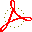## Lecture 4 - Dynamical theory of turbulence

As a turbulent flow evolves, every so often we catch a glimpse of a familiar pattern. For any finite spatial resolution, the system follows approximately for a finite time a pattern belonging to a finite alphabet of admissible patterns. The long term dynamics is a walk through the space of such unstable patterns.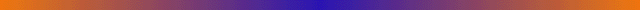## Index, ChaosBook.org## Appendix A - Brief history of chaos

Classical mechanics has not stood still since Newton. The formalism that we use today was developed by Euler and Lagrange. By the end of the 1800's the three problems that would lead to the notion of chaotic dynamics were already known: the three-body problem, the ergodic hypothesis, and nonlinear oscillators.Excerpted from ChaosBook.org/version11,   28 dec 2004;
please cite the latest stable edition
icons explained: